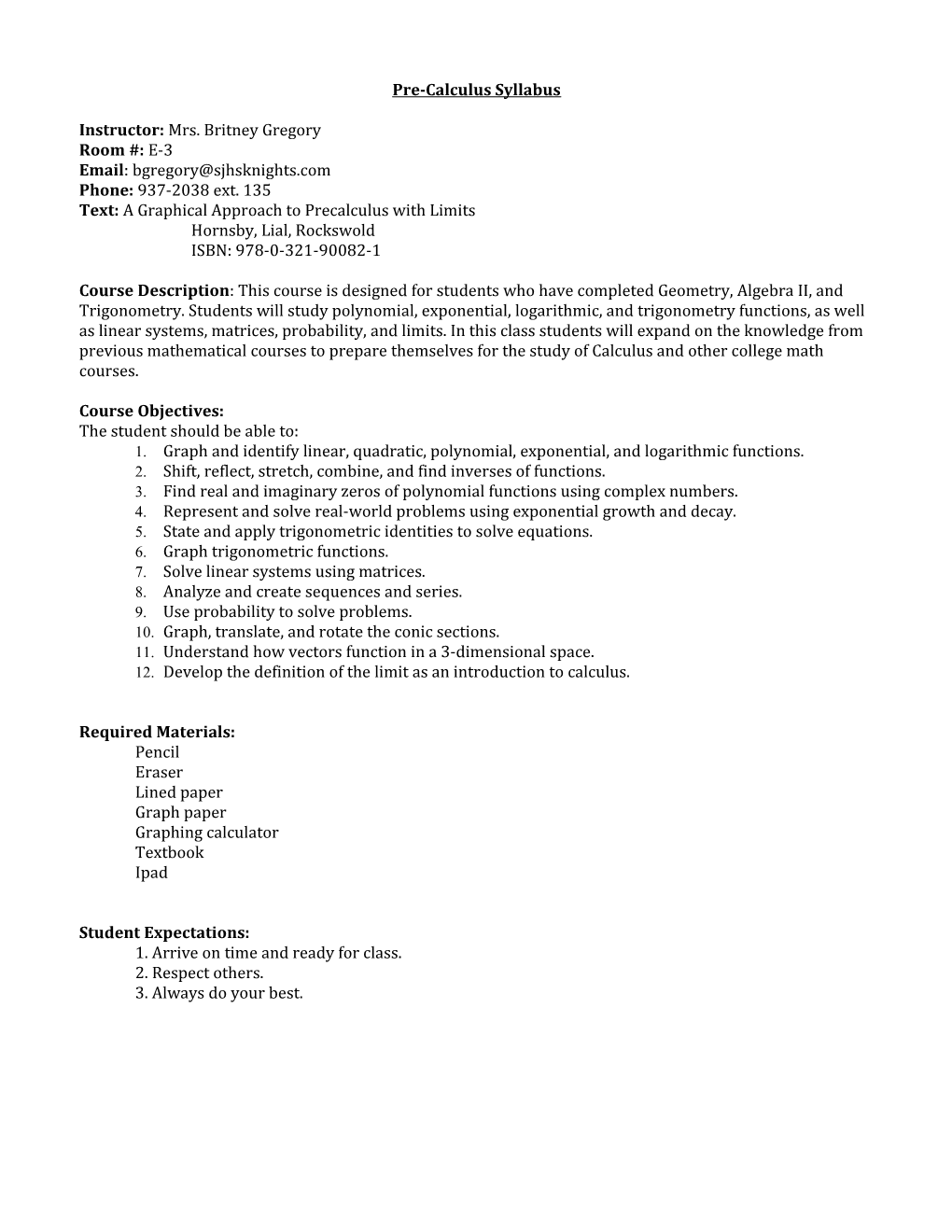# Pre-Calculus SyllabusPre-Calculus Syllabus

Instructor: Mrs. Britney Gregory

Room #:E-3

Email:

Phone:937-2038 ext. 135

Text:A Graphical Approach to Precalculus with Limits

Hornsby, Lial, Rockswold

ISBN: 978-0-321-90082-1

Course Description: This course is designed for students who have completed Geometry, Algebra II, and Trigonometry. Students will study polynomial, exponential, logarithmic, and trigonometry functions, as well as linear systems, matrices, probability, and limits. In this class students will expand on the knowledge from previous mathematical courses to prepare themselves for the study of Calculus and other college math courses.

Course Objectives:

The student should be able to:

1. Graph and identify linear, quadratic, polynomial, exponential, and logarithmic functions.
2. Shift, reflect, stretch, combine, and find inverses of functions.
3. Find real and imaginary zeros of polynomial functions using complex numbers.
4. Represent and solve real-world problems using exponential growth and decay.
5. State and apply trigonometric identities to solve equations.
6. Graph trigonometric functions.
7. Solve linear systems using matrices.
8. Analyze and create sequences and series.
9. Use probability to solve problems.
10. Graph, translate, and rotate the conic sections.
11. Understand how vectors function in a 3-dimensional space.
12. Develop the definition of the limit as an introduction to calculus.

Required Materials:

Pencil

Eraser

Lined paper

Graph paper

Graphing calculator

Textbook

Student Expectations:

1. Arrive on time and ready for class.

2. Respect others.

Classroom Policies and Procedures:

1.Homework will be collected on a daily basis.

2. The beginning of each class period will be designated to answering any questions students had when completing the homework assignment.

3. At the end of the period, students will wait until the teacher dismisses them to leave the classroom.

4. Students will clean up personal work area before leaving class.

5. ​No hats, hoodies or sunglasses are to be worn in the classroom.

​6. No food or beverages are allowed in the classroom.

Students’ grades will be determined by homework, classwork, chapter assessments, and participation.

Homework & Classwork: 30%

Chapter Assessments: 40%

Final: 20%

Participation: 10%

Homework: All homework assignments will contain a list of required problems and a list of suggested problems. Required problems will be due the day after the completion of a section. Suggested problems are optional study tools and maybe turned in on assessment days for up to 5% extra credit on a test.

Late work: Late work will only be accepted in the case of an excused absence and all work will be due when the student returns to class. All assignments can be found on the school website.

Make-up Tests/Quizzes: If a student is absent on the day of a test or quiz the student will be expected to schedule a retake with the teacher upon the students return to class and must be completed no later than one week after original test/quiz was given. If a student is absent for more than a week, please meet with teacher to create an individualized plan.

98.5 to 100 = A+

91.5 to 98.4 = A

89.5 to 91.4 = A-

87.5 to 89.4 = B+

81.5 to 87.4 = B

79.5 to 81.4 = B-

77.5 to 79.4 = C+

71.5 to 77.4 = C

69.5 to 71.4 = C-

67.5 to 69.4 = D+

61.5 to 67.4 = D

59.5 to 61.4 = D-

Below 59.4 = F

Student and parent/guardian should review syllabus together, sign, and return to teacher.

______

Student SignatureParent Signature